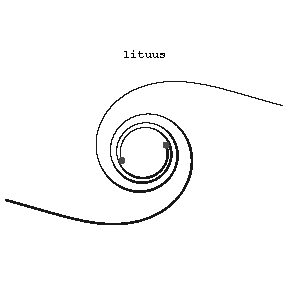# Lituus

Jump to: navigation, search

A plane transcendental curve whose equation in polar coordinates is

$$\rho=\frac{a}{\sqrt\phi}.$$Figure: l059750a

To every value of $\phi$ correspond two values of $\rho$, one positive and one negative. The curve consists of two branches, that both approach the pole asymptotically (see Fig.). The line $\phi=0,\phi=\pi$ is an asymptote at $\rho=\pm\infty$, and $(1/2,a\sqrt2)$ and $(-1/2,-a\sqrt2)$ are points of inflection. The lituus is related to the so-called algebraic spirals.